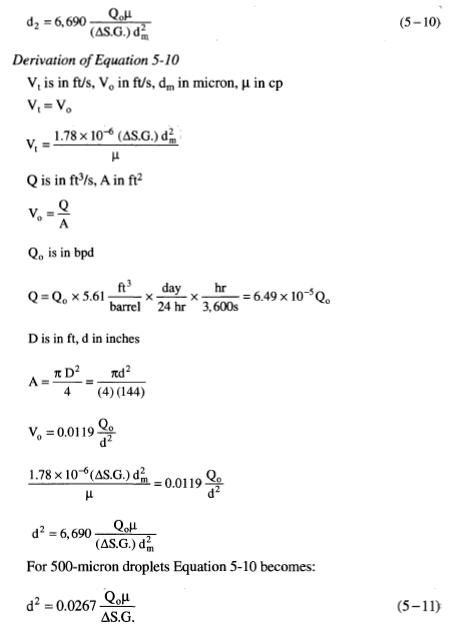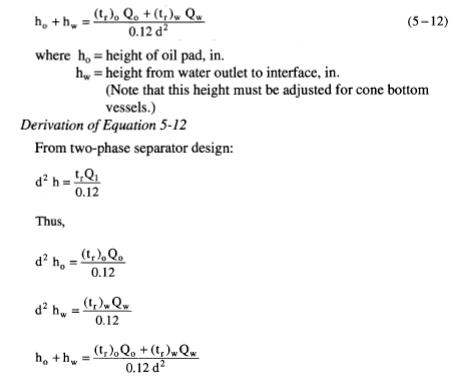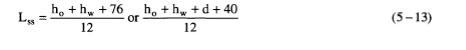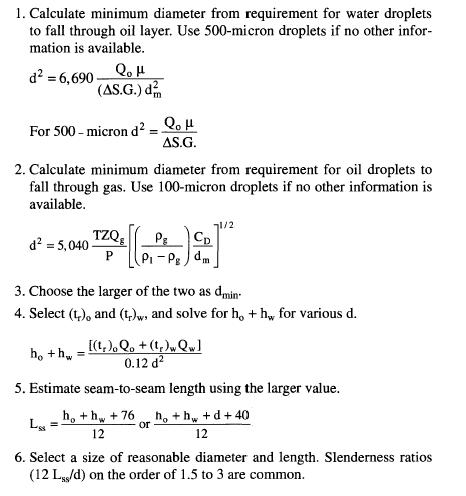As with vertical two-phase separators, a minimum diameter must be  maintained to assure adequate gas capacity. In addition, vertical threephase  separators must maintain a minimum diameter to allow the 500 micron water droplets to settle. The height of the three-phase separator is  determined from retention time considerations.

Gas Capacity
The gas capacity constraints provide the following formulaSettling
The requirement for settling water droplets from the oil requires that the following equation must be satisfied:Retention TimeSeam-to-Seam Length and Slenderness Ratios
As in the case of a vertical two-phase separator, the seam-to-seam  length (Lss) can be approximated from the geometry once h0 and hw are  chosen. For screening purposes it can be assumed that Lss is the larger of  the two values in the following equations:where d is the minimum diameter for gas capacity.
Any d larger than that calculated by Equation 5-9 and 5-10 and that  satisfies Equation 5-12 is acceptable. Diameter should be chosen with  slenderness ratios less than 4. Most vertical three-phase separators have slenderness ratios on the order of 1.5 to 3 to keep within reasonable height restrictions.

Procedure for Sizing Three-Phase, Vertical Separators